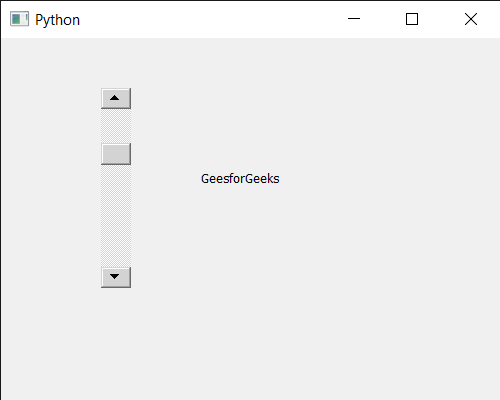# PyQt5 QScrollBar – Setting Value

• Last Updated : 04 Aug, 2021

In this article we will see how we can set the value of QScrollBar. QScrollBar is a control that enables the user to access parts of a document that is larger than the widget used to display it. Slider is the scroll-able object inside the bar. Value is basically depend upon the slider position, scroll bar value changes according to the position of the slider, value increases when slider goes down and decreases when slider moves up.

In order to do this we will use setValue method with the scroll bar object.
Syntax : scroll.setValue(n)
Argument : It takes integer as argument
Return : It returns None

Example : Below is the implementation

## Python3

 `# importing libraries``from` `PyQt5.QtWidgets ``import` `*``from` `PyQt5 ``import` `QtCore, QtGui``from` `PyQt5.QtGui ``import` `*``from` `PyQt5.QtCore ``import` `*``import` `sys`  `class` `Window(QMainWindow):` `    ``def` `__init__(``self``):``        ``super``().__init__()` `        ``# setting title``        ``self``.setWindowTitle(``"Python "``)` `        ``# setting geometry``        ``self``.setGeometry(``100``, ``100``, ``500``, ``400``)` `        ``# calling method``        ``self``.UiComponents()` `        ``# showing all the widgets``        ``self``.show()`   `    ``# method for components``    ``def` `UiComponents(``self``):` `        ``scroll ``=` `QScrollBar(``self``)` `        ``# setting geometry of the scroll bar``        ``scroll.setGeometry(``100``, ``50``, ``30``, ``200``)` `        ``# setting value of scroll bar``        ``scroll.setValue(``25``)` `        ``# making its background color to green``        ``scroll.setStyleSheet(``"background : lightgrey;"``)` `        ``# creating a label``        ``label ``=` `QLabel(``"GeesforGeeks"``, ``self``)` `        ``# setting geometry to the label``        ``label.setGeometry(``200``, ``100``, ``300``, ``80``)` `        ``# making label multi line``        ``label.setWordWrap(``True``)`  `# create pyqt5 app``App ``=` `QApplication(sys.argv)` `# create the instance of our Window``window ``=` `Window()` `# start the app``sys.exit(App.``exec``())`

Output :My Personal Notes arrow_drop_up